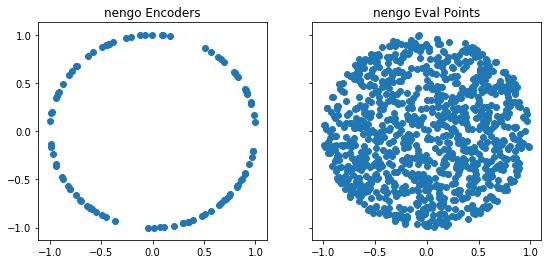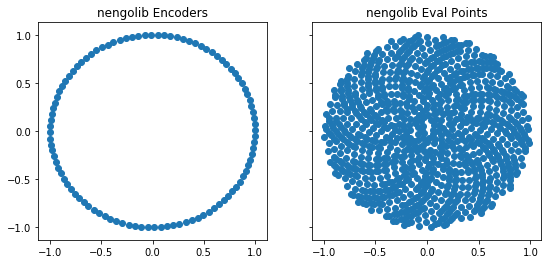# Network Improvements¶

The class nengolib.Network() is intended to serve as a drop-in replacement for nengo.Network(). This new class:

• samples encoders more uniformly;
• samples eval_points more uniformly; and
• uses neurons which spike at the ideal rate regardless of dt (see Nengo #975; default in nengo>=2.1.1).

As a result, the performance of ensembles should increase, both in terms of their representational quality (the encoders become better “representatives”) and the generalization error from the decoders (their approximation error on unseen test points). A current limitation is that the improvement only occurs for up to 40-dimensional ensembles (beyond this the original implementation is used).

:

%pylab inline
import pylab
try:
import seaborn as sns  # optional; prettier graphs
except ImportError:
pass

import nengo
import nengolib

def plot_points(module, n_neurons=100):
with module.Network() as model:
x = nengo.Ensemble(n_neurons, 2)
nengo.Connection(x, nengo.Node(size_in=2))
sim = nengo.Simulator(model)

fig, ax = pylab.subplots(1, 2, sharey=True, figsize=(9, 4))
ax.scatter(*sim.data[x].encoders.T)
ax.scatter(*sim.data[x].eval_points.T)
module = module.__name__.split('.', 1)
ax.set_title('%s Encoders' % module)
ax.set_title('%s Eval Points' % module)
pylab.show()

plot_points(nengo)
plot_points(nengolib)

Populating the interactive namespace from numpy and matplotlib

0%0%: